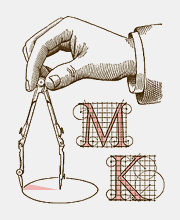Rhombi 4

Inscribe a rhombus into the given parallelogram so that one of its vertices is A and each side of the parallelogram contains one vertex. To draw the rhombus, use the Polygon tool.

The better of two solutions is the one with the smaller number of new lines. If two solutions have equally many new lines, the numbers of new points are compared.